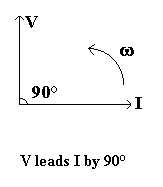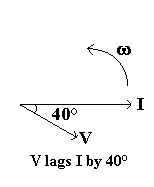# Phasor Diagrams

Phasor diagrams can be used to represent voltages and currents in A.C. circuits. They show not only the magnitude (size) of the voltage or current, but also the phase angle of it.

See the diagrams below for some examples. Diagrams for components in series are drawn using the current as the reference phasor (since it is the same for all the components in series), those for parallel circuits use the voltage as the reference phasor.

The length of the line for each component represents the magnitude of the voltage or current, and the angle between it and the reference phasor is the phase angle.

Note that often phasor diagrams are only sketched, rather than drawn to scale!

#### Example: